# 10.4 Polar coordinates: graphs  (Page 7/16)

 Page 7 / 16

Sketch the graph of $\text{\hspace{0.17em}}r=-\theta \text{\hspace{0.17em}}$ over the interval $\text{\hspace{0.17em}}\left[0,4\pi \right].$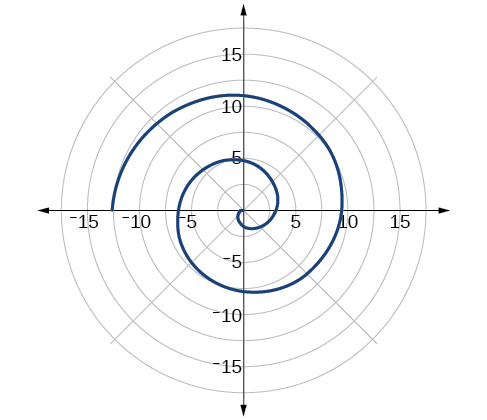## Summary of curves

We have explored a number of seemingly complex polar curves in this section. [link] and [link] summarize the graphs and equations for each of these curves.

Access these online resources for additional instruction and practice with graphs of polar coordinates.

## Key concepts

• It is easier to graph polar equations if we can test the equations for symmetry with respect to the line $\text{\hspace{0.17em}}\theta =\frac{\pi }{2},\text{\hspace{0.17em}}$ the polar axis, or the pole.
• There are three symmetry tests that indicate whether the graph of a polar equation will exhibit symmetry. If an equation fails a symmetry test, the graph may or may not exhibit symmetry. See [link] .
• Polar equations may be graphed by making a table of values for $\text{\hspace{0.17em}}\theta \text{\hspace{0.17em}}$ and $\text{\hspace{0.17em}}r.$
• The maximum value of a polar equation is found by substituting the value $\text{\hspace{0.17em}}\theta \text{\hspace{0.17em}}$ that leads to the maximum value of the trigonometric expression.
• The zeros of a polar equation are found by setting $\text{\hspace{0.17em}}r=0\text{\hspace{0.17em}}$ and solving for $\text{\hspace{0.17em}}\theta .\text{\hspace{0.17em}}$ See [link] .
• Some formulas that produce the graph of a circle in polar coordinates are given by $\text{\hspace{0.17em}}r=a\mathrm{cos}\text{\hspace{0.17em}}\theta \text{\hspace{0.17em}}$ and $\text{\hspace{0.17em}}r=a\mathrm{sin}\text{\hspace{0.17em}}\theta .\text{\hspace{0.17em}}$ See [link] .
• The formulas that produce the graphs of a cardioid are given by $\text{\hspace{0.17em}}r=a±b\mathrm{cos}\text{\hspace{0.17em}}\theta \text{\hspace{0.17em}}$ and $\text{\hspace{0.17em}}r=a±b\mathrm{sin}\text{\hspace{0.17em}}\theta ,\text{\hspace{0.17em}}$ for $\text{\hspace{0.17em}}a>0,\text{\hspace{0.17em}}\text{\hspace{0.17em}}b>0,\text{\hspace{0.17em}}$ and $\text{\hspace{0.17em}}\frac{a}{b}=1.\text{\hspace{0.17em}}$ See [link] .
• The formulas that produce the graphs of a one-loop limaçon are given by $\text{\hspace{0.17em}}r=a±b\mathrm{cos}\text{\hspace{0.17em}}\theta \text{\hspace{0.17em}}$ and $\text{\hspace{0.17em}}r=a±b\mathrm{sin}\text{\hspace{0.17em}}\theta \text{\hspace{0.17em}}$ for $\text{\hspace{0.17em}}1<\frac{a}{b}<2.\text{\hspace{0.17em}}$ See [link] .
• The formulas that produce the graphs of an inner-loop limaçon are given by $\text{\hspace{0.17em}}r=a±b\mathrm{cos}\text{\hspace{0.17em}}\theta \text{\hspace{0.17em}}$ and $\text{\hspace{0.17em}}r=a±b\mathrm{sin}\text{\hspace{0.17em}}\theta \text{\hspace{0.17em}}$ for $\text{\hspace{0.17em}}a>0,\text{\hspace{0.17em}}\text{\hspace{0.17em}}b>0,\text{\hspace{0.17em}}$ and $\text{\hspace{0.17em}}a See [link] .
• The formulas that produce the graphs of a lemniscates are given by $\text{\hspace{0.17em}}{r}^{2}={a}^{2}\mathrm{cos}\text{\hspace{0.17em}}2\theta \text{\hspace{0.17em}}$ and $\text{\hspace{0.17em}}{r}^{2}={a}^{2}\mathrm{sin}\text{\hspace{0.17em}}2\theta ,\text{\hspace{0.17em}}$ where $\text{\hspace{0.17em}}a\ne 0.$ See [link] .
• The formulas that produce the graphs of rose curves are given by $\text{\hspace{0.17em}}r=a\mathrm{cos}\text{\hspace{0.17em}}n\theta \text{\hspace{0.17em}}$ and $\text{\hspace{0.17em}}r=a\mathrm{sin}\text{\hspace{0.17em}}n\theta ,\text{\hspace{0.17em}}$ where $\text{\hspace{0.17em}}a\ne 0;\text{\hspace{0.17em}}$ if $\text{\hspace{0.17em}}n\text{\hspace{0.17em}}$ is even, there are $\text{\hspace{0.17em}}2n\text{\hspace{0.17em}}$ petals, and if $\text{\hspace{0.17em}}n\text{\hspace{0.17em}}$ is odd, there are $\text{\hspace{0.17em}}n\text{\hspace{0.17em}}$ petals. See [link] and [link] .
• The formula that produces the graph of an Archimedes’ spiral is given by $\text{\hspace{0.17em}}r=\theta ,\text{\hspace{0.17em}}\text{\hspace{0.17em}}\theta \ge 0.\text{\hspace{0.17em}}$ See [link] .

## Verbal

Describe the three types of symmetry in polar graphs, and compare them to the symmetry of the Cartesian plane.

Symmetry with respect to the polar axis is similar to symmetry about the $\text{\hspace{0.17em}}x$ -axis, symmetry with respect to the pole is similar to symmetry about the origin, and symmetric with respect to the line $\text{\hspace{0.17em}}\theta =\frac{\pi }{2}\text{\hspace{0.17em}}$ is similar to symmetry about the $\text{\hspace{0.17em}}y$ -axis.

Which of the three types of symmetries for polar graphs correspond to the symmetries with respect to the x -axis, y -axis, and origin?

What are the steps to follow when graphing polar equations?

Test for symmetry; find zeros, intercepts, and maxima; make a table of values. Decide the general type of graph, cardioid, limaçon, lemniscate, etc., then plot points at and sketch the graph.

Describe the shapes of the graphs of cardioids, limaçons, and lemniscates.

What part of the equation determines the shape of the graph of a polar equation?

The shape of the polar graph is determined by whether or not it includes a sine, a cosine, and constants in the equation.

## Graphical

For the following exercises, test the equation for symmetry.

$r=5\mathrm{cos}\text{\hspace{0.17em}}3\theta$

$r=3-3\mathrm{cos}\text{\hspace{0.17em}}\theta$

symmetric with respect to the polar axis

$r=3+2\mathrm{sin}\text{\hspace{0.17em}}\theta$

$r=3\mathrm{sin}\text{\hspace{0.17em}}2\theta$

symmetric with respect to the polar axis, symmetric with respect to the line $\theta =\frac{\pi }{2},$ symmetric with respect to the pole

$r=4$

$r=2\theta$

no symmetry

$r=4\mathrm{cos}\text{\hspace{0.17em}}\frac{\theta }{2}$

$r=\frac{2}{\theta }$

no symmetry

$r=3\sqrt{1-{\mathrm{cos}}^{2}\theta }$

$r=\sqrt{5\mathrm{sin}\text{\hspace{0.17em}}2\theta }$

symmetric with respect to the pole

For the following exercises, graph the polar equation. Identify the name of the shape.

$r=3\mathrm{cos}\text{\hspace{0.17em}}\theta$

$r=4\mathrm{sin}\text{\hspace{0.17em}}\theta$

circle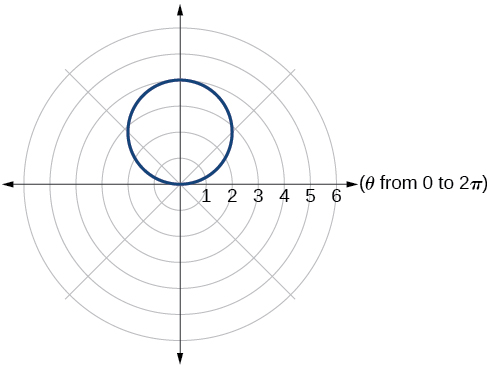$r=2+2\mathrm{cos}\text{\hspace{0.17em}}\theta$

$r=2-2\mathrm{cos}\text{\hspace{0.17em}}\theta$

cardioid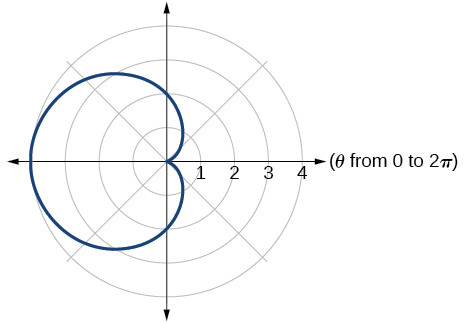$r=5-5\mathrm{sin}\text{\hspace{0.17em}}\theta$

$r=3+3\mathrm{sin}\text{\hspace{0.17em}}\theta$

cardioid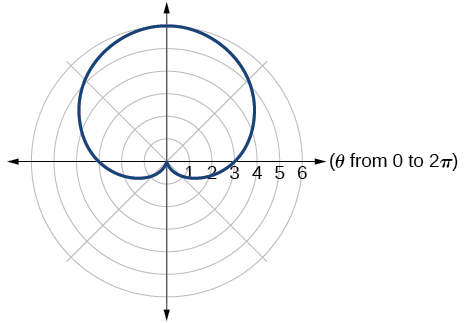$r=3+2\mathrm{sin}\text{\hspace{0.17em}}\theta$

$r=7+4\mathrm{sin}\text{\hspace{0.17em}}\theta$

one-loop/dimpled limaçon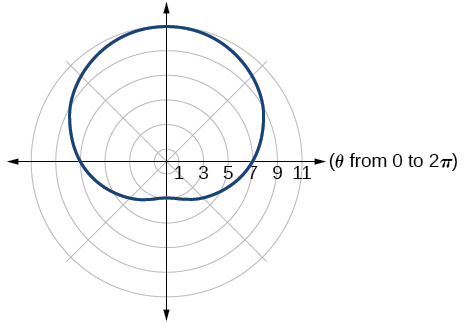$r=4+3\mathrm{cos}\text{\hspace{0.17em}}\theta$

$r=5+4\mathrm{cos}\text{\hspace{0.17em}}\theta$

one-loop/dimpled limaçon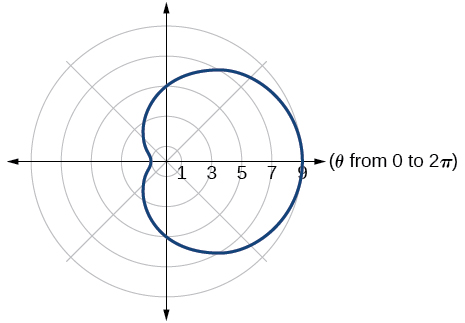$r=10+9\mathrm{cos}\text{\hspace{0.17em}}\theta$

$r=1+3\mathrm{sin}\text{\hspace{0.17em}}\theta$

inner loop/two-loop limaçon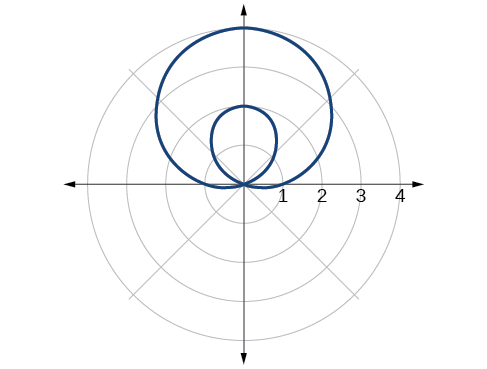$r=2+5\mathrm{sin}\text{\hspace{0.17em}}\theta$

$r=5+7\mathrm{sin}\text{\hspace{0.17em}}\theta$

inner loop/two-loop limaçon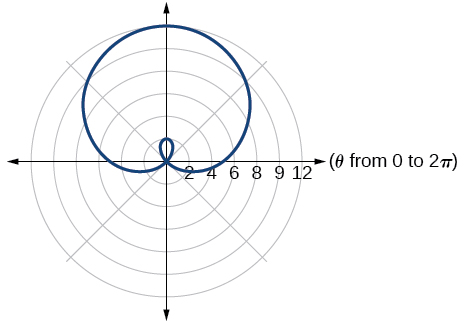$r=2+4\mathrm{cos}\text{\hspace{0.17em}}\theta$

$r=5+6\mathrm{cos}\text{\hspace{0.17em}}\theta$

inner loop/two-loop limaçon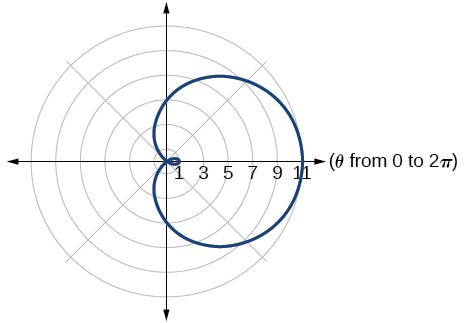${r}^{2}=36\mathrm{cos}\left(2\theta \right)$

${r}^{2}=10\mathrm{cos}\left(2\theta \right)$

lemniscate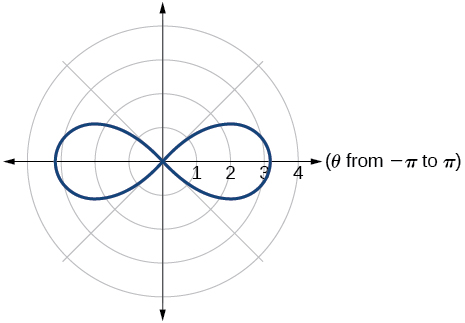${r}^{2}=4\mathrm{sin}\left(2\theta \right)$

${r}^{2}=10\mathrm{sin}\left(2\theta \right)$

lemniscate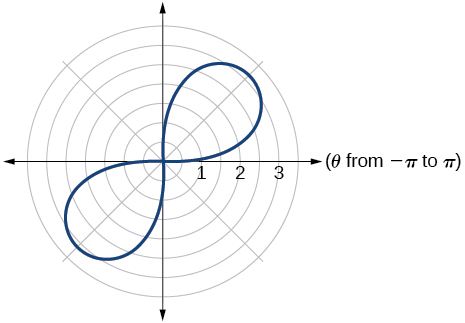$r=3\text{sin}\left(2\theta \right)$

$r=3\text{cos}\left(2\theta \right)$

rose curve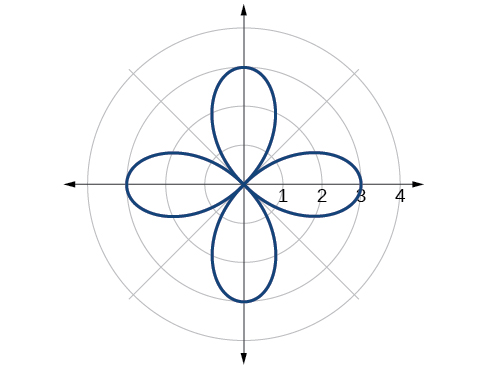$r=5\text{sin}\left(3\theta \right)$

$r=4\text{sin}\left(4\theta \right)$

rose curve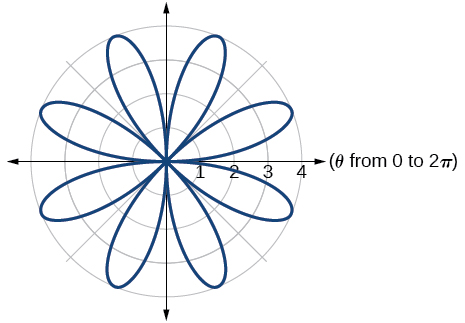$r=4\text{sin}\left(5\theta \right)$

$r=-\theta$

Archimedes’ spiral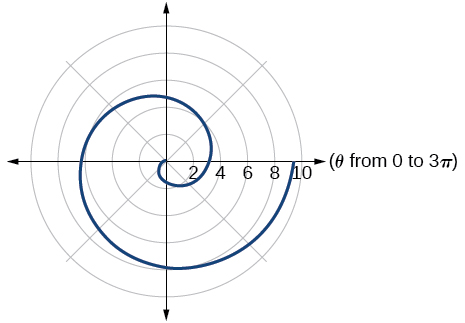$r=2\theta$

$r=-3\theta$

Archimedes’ spiral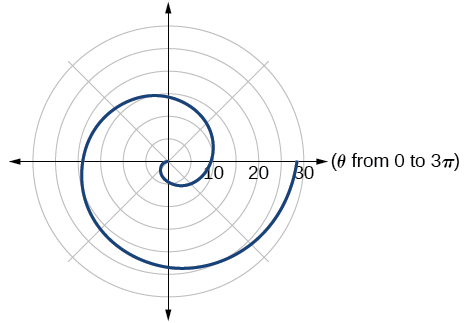## Technology

For the following exercises, use a graphing calculator to sketch the graph of the polar equation.

$r=\frac{1}{\theta }$

$r=\frac{1}{\sqrt{\theta }}$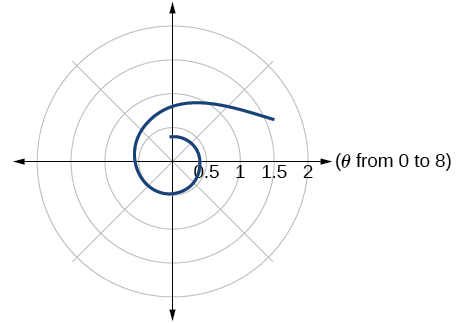$r=2\mathrm{sin}\text{\hspace{0.17em}}\theta \mathrm{tan}\text{\hspace{0.17em}}\theta ,$ a cissoid

$r=2\sqrt{1-{\mathrm{sin}}^{2}\theta }$ , a hippopede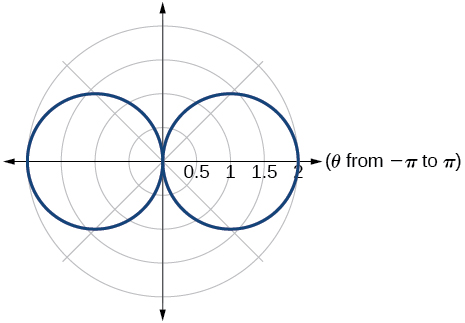$r=5+\mathrm{cos}\left(4\theta \right)$

$r=2-\mathrm{sin}\left(2\theta \right)$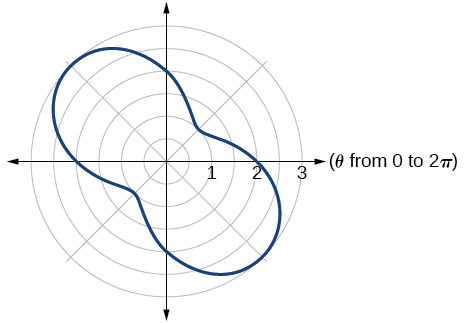$r={\theta }^{2}$

$r=\theta +1$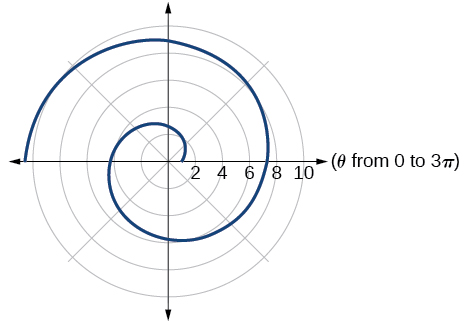$r=\theta \mathrm{sin}\text{\hspace{0.17em}}\theta$

$r=\theta \mathrm{cos}\text{\hspace{0.17em}}\theta$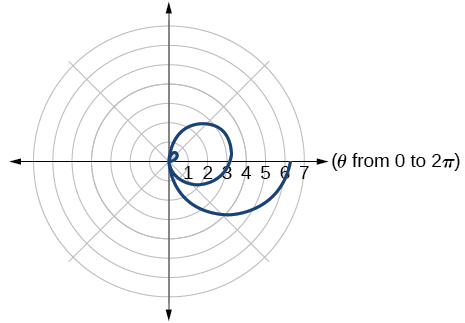For the following exercises, use a graphing utility to graph each pair of polar equations on a domain of $\text{\hspace{0.17em}}\left[0,4\pi \right]\text{\hspace{0.17em}}$ and then explain the differences shown in the graphs.

$r=\theta ,r=-\theta$

$r=\theta ,r=\theta +\mathrm{sin}\text{\hspace{0.17em}}\theta$

They are both spirals, but not quite the same.

$r=\mathrm{sin}\text{\hspace{0.17em}}\theta +\theta ,r=\mathrm{sin}\text{\hspace{0.17em}}\theta -\theta$

$r=2\mathrm{sin}\left(\frac{\theta }{2}\right),r=\theta \mathrm{sin}\left(\frac{\theta }{2}\right)$

Both graphs are curves with 2 loops. The equation with a coefficient of $\text{\hspace{0.17em}}\theta \text{\hspace{0.17em}}$ has two loops on the left, the equation with a coefficient of 2 has two loops side by side. Graph these from 0 to $\text{\hspace{0.17em}}4\pi \text{\hspace{0.17em}}$ to get a better picture.

$r=\mathrm{sin}\left(\mathrm{cos}\left(3\theta \right)\right)\text{\hspace{0.17em}}\text{\hspace{0.17em}}r=\mathrm{sin}\left(3\theta \right)$

On a graphing utility, graph $\text{\hspace{0.17em}}r=\mathrm{sin}\left(\frac{16}{5}\theta \right)\text{\hspace{0.17em}}$ on $\text{\hspace{0.17em}}\left[0,4\pi \right],\left[0,8\pi \right],\left[0,12\pi \right],\text{\hspace{0.17em}}$ and $\text{\hspace{0.17em}}\left[0,16\pi \right].\text{\hspace{0.17em}}$ Describe the effect of increasing the width of the domain.

When the width of the domain is increased, more petals of the flower are visible.

On a graphing utility, graph and sketch $\text{\hspace{0.17em}}r=\mathrm{sin}\text{\hspace{0.17em}}\theta +{\left(\mathrm{sin}\left(\frac{5}{2}\theta \right)\right)}^{3}\text{\hspace{0.17em}}$ on $\text{\hspace{0.17em}}\left[0,4\pi \right].$

On a graphing utility, graph each polar equation. Explain the similarities and differences you observe in the graphs.

$\begin{array}{l}\begin{array}{l}\\ {r}_{1}=3\mathrm{sin}\left(3\theta \right)\end{array}\hfill \\ {r}_{2}=2\mathrm{sin}\left(3\theta \right)\hfill \\ {r}_{3}=\mathrm{sin}\left(3\theta \right)\hfill \end{array}$

The graphs are three-petal, rose curves. The larger the coefficient, the greater the curve’s distance from the pole.

On a graphing utility, graph each polar equation. Explain the similarities and differences you observe in the graphs.

$\begin{array}{l}\begin{array}{l}\\ {r}_{1}=3+3\mathrm{cos}\text{\hspace{0.17em}}\theta \end{array}\hfill \\ {r}_{2}=2+2\mathrm{cos}\text{\hspace{0.17em}}\theta \hfill \\ {r}_{3}=1+\mathrm{cos}\text{\hspace{0.17em}}\theta \hfill \end{array}$

On a graphing utility, graph each polar equation. Explain the similarities and differences you observe in the graphs.

$\begin{array}{l}\begin{array}{l}\\ {r}_{1}=3\theta \end{array}\hfill \\ {r}_{2}=2\theta \hfill \\ {r}_{3}=\theta \hfill \end{array}$

The graphs are spirals. The smaller the coefficient, the tighter the spiral.

## Extensions

For the following exercises, draw each polar equation on the same set of polar axes, and find the points of intersection.

${r}_{1}=3+2\mathrm{sin}\text{\hspace{0.17em}}\theta ,\text{\hspace{0.17em}}{r}_{2}=2$

${r}_{1}=6-4\mathrm{cos}\text{\hspace{0.17em}}\theta ,\text{\hspace{0.17em}}{r}_{2}=4$

$\left(4,\frac{\pi }{3}\right),\left(4,\frac{5\pi }{3}\right)$

${r}_{1}=1+\mathrm{sin}\text{\hspace{0.17em}}\theta ,\text{\hspace{0.17em}}{r}_{2}=3\mathrm{sin}\text{\hspace{0.17em}}\theta$

${r}_{1}=1+\mathrm{cos}\text{\hspace{0.17em}}\theta ,\text{\hspace{0.17em}}{r}_{2}=3\mathrm{cos}\text{\hspace{0.17em}}\theta$

$\left(\frac{3}{2},\frac{\pi }{3}\right),\left(\frac{3}{2},\frac{5\pi }{3}\right)$

${r}_{1}=\mathrm{cos}\left(2\theta \right),\text{\hspace{0.17em}}{r}_{2}=\mathrm{sin}\left(2\theta \right)$

${r}_{1}={\mathrm{sin}}^{2}\left(2\theta \right),\text{\hspace{0.17em}}{r}_{2}=1-\mathrm{cos}\left(4\theta \right)$

$\left(0,\frac{\pi }{2}\right),\text{\hspace{0.17em}}\left(0,\pi \right),\text{\hspace{0.17em}}\left(0,\frac{3\pi }{2}\right),\text{\hspace{0.17em}}\left(0,2\pi \right)$

${r}_{1}=\sqrt{3},\text{\hspace{0.17em}}{r}_{2}=2\mathrm{sin}\left(\theta \right)$

${r}_{1}{}^{2}=\mathrm{sin}\text{\hspace{0.17em}}\theta ,{r}_{2}{}^{2}=\mathrm{cos}\text{\hspace{0.17em}}\theta$

$\left(\frac{\sqrt{8}}{2},\frac{\pi }{4}\right),\text{\hspace{0.17em}}\left(\frac{\sqrt{8}}{2},\frac{5\pi }{4}\right)\text{\hspace{0.17em}}$ and at $\text{\hspace{0.17em}}\theta =\frac{3\pi }{4},\text{\hspace{0.17em}}\text{\hspace{0.17em}}\frac{7\pi }{4}\text{\hspace{0.17em}}\text{\hspace{0.17em}}$ since $\text{\hspace{0.17em}}r\text{\hspace{0.17em}}$ is squared

${r}_{1}=1+\mathrm{cos}\text{\hspace{0.17em}}\theta ,\text{\hspace{0.17em}}{r}_{2}=1-\mathrm{sin}\text{\hspace{0.17em}}\theta$

(1+cosA+IsinA)(1+cosB+isinB)/(cos@+isin@)(cos$+isin$)
hatdog
Mark
how we can draw three triangles of distinctly different shapes. All the angles will be cutt off each triangle and placed side by side with vertices touching
bsc F. y algebra and trigonometry pepper 2
given that x= 3/5 find sin 3x
4
DB
remove any signs and collect terms of -2(8a-3b-c)
-16a+6b+2c
Will
Joeval
(x2-2x+8)-4(x2-3x+5)
sorry
Miranda
x²-2x+9-4x²+12x-20 -3x²+10x+11
Miranda
x²-2x+9-4x²+12x-20 -3x²+10x+11
Miranda
(X2-2X+8)-4(X2-3X+5)=0 ?
master
The anwser is imaginary number if you want to know The anwser of the expression you must arrange The expression and use quadratic formula To find the answer
master
The anwser is imaginary number if you want to know The anwser of the expression you must arrange The expression and use quadratic formula To find the answer
master
Y
master
master
Soo sorry (5±Root11* i)/3
master
Mukhtar
2x²-6x+1=0
Ife
explain and give four example of hyperbolic function
What is the correct rational algebraic expression of the given "a fraction whose denominator is 10 more than the numerator y?
y/y+10
Mr
Find nth derivative of eax sin (bx + c).
Find area common to the parabola y2 = 4ax and x2 = 4ay.
Anurag
y2=4ax= y=4ax/2. y=2ax
akash
A rectangular garden is 25ft wide. if its area is 1125ft, what is the length of the garden
to find the length I divide the area by the wide wich means 1125ft/25ft=45
Miranda
thanks
Jhovie
What do you call a relation where each element in the domain is related to only one value in the range by some rules?
A banana.
Yaona
given 4cot thither +3=0and 0°<thither <180° use a sketch to determine the value of the following a)cos thither
what are you up to?
nothing up todat yet
Miranda
hi
jai
hello
jai
Miranda Drice
jai
aap konsi country se ho
jai
which language is that
Miranda
I am living in india
jai
good
Miranda
what is the formula for calculating algebraic
I think the formula for calculating algebraic is the statement of the equality of two expression stimulate by a set of addition, multiplication, soustraction, division, raising to a power and extraction of Root. U believe by having those in the equation you will be in measure to calculate it
Miranda
state and prove Cayley hamilton therom
hello
Propessor
hi
Miranda
the Cayley hamilton Theorem state if A is a square matrix and if f(x) is its characterics polynomial then f(x)=0 in another ways evey square matrix is a root of its chatacteristics polynomial.
Miranda
hi
jai
hi Miranda
jai
thanks
Propessor
welcome
jai

#### Get Jobilize Job Search Mobile App in your pocket Now!ByByBy Charles JumperBy Janet ForresterBy Maureen MillerBy OpenStaxBy OpenStaxBy Steve GibbsBy JavaChamp TeamBy OpenStaxBy OpenStaxBy